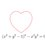# The Third Indian SAT+SMT School

I attended The Third Indian SAT+SMT School held at IIIT Hyderabad, this December.

SAT is short for boolean satisfiability. Consider the boolean formula $$p \land \neg p$$. This formula will never be satisfied, regardless of whether $$p$$ is true or not. However, if you consider the formula $$p \lor q$$, it can be satisfied by setting either $$p$$ or $$q$$ to true. The question is, Can we devise a method which when given an arbitrary boolean formula decides if it is satisfiable?. SMT (Satisfiability Modulo Theories) is a related question where instead of having just $p$ or $q$ or propositional letters, we have more complex objects like linear arithmetic formulae ($x + y > 7$, $x = 2y$, etc) or statements about an array (a[i]=b[j], etc), etc. As Vijay Ganesh says, "SMT Solvers are like uber SAT solvers".

The SAT problem is very interesting for Computer Scientists because an efficient solution to this problem would give us an efficient solution to a large class of interesting problems. This is known as the P vs NP problem. In a rather informal sense, this would mean that any problem whose solution can be verified easily can also be solved easily, which would be a rather surprising result!

Nonetheless, the interesting part is that although the theory suggests that these problems should be very hard; in practice, we solve SAT problems with thousands of variables in a matter of seconds for industrial purposes everyday. These techniques are useful for a number of real life applications. Here is a quote from the SAT+SMT website

SAT and SMT solvers are the backbone of a wide range of academic and industrial R&D activities today. These include software and hardware verification, logistics, planning, operations research, non-linear discrete optimization, model counting, etc. Recent developments in the field suggest that these solvers may soon be leveraged in an even wider range of applications that touch almost all aspects of computing. Unfortunately, in India, the technical study of these solvers is limited to a few individuals/groups. This has hampered the growth of research and development in this area, both in the Indian academia and in the Indian industry. Keeping in view of this gap, we are organizing a winter school series on SAT+SMT solvers.

If you wish to check out an online course related to practical applications of such technology, check out this course on Model Checking2 years, 7 months ago

This discussion board is a place to discuss our Daily Challenges and the math and science related to those challenges. Explanations are more than just a solution — they should explain the steps and thinking strategies that you used to obtain the solution. Comments should further the discussion of math and science.

When posting on Brilliant:

• Use the emojis to react to an explanation, whether you're congratulating a job well done , or just really confused .
• Ask specific questions about the challenge or the steps in somebody's explanation. Well-posed questions can add a lot to the discussion, but posting "I don't understand!" doesn't help anyone.
• Try to contribute something new to the discussion, whether it is an extension, generalization or other idea related to the challenge.

MarkdownAppears as
*italics* or _italics_ italics
**bold** or __bold__ bold
- bulleted- list
• bulleted
• list
1. numbered2. list
1. numbered
2. list
Note: you must add a full line of space before and after lists for them to show up correctly
paragraph 1paragraph 2

paragraph 1

paragraph 2

[example link](https://brilliant.org)example link
> This is a quote
This is a quote
    # I indented these lines
# 4 spaces, and now they show
# up as a code block.

print "hello world"
# I indented these lines
# 4 spaces, and now they show
# up as a code block.

print "hello world"
MathAppears as
Remember to wrap math in $$ ... $$ or $ ... $ to ensure proper formatting.
2 \times 3 $2 \times 3$
2^{34} $2^{34}$
a_{i-1} $a_{i-1}$
\frac{2}{3} $\frac{2}{3}$
\sqrt{2} $\sqrt{2}$
\sum_{i=1}^3 $\sum_{i=1}^3$
\sin \theta $\sin \theta$
\boxed{123} $\boxed{123}$